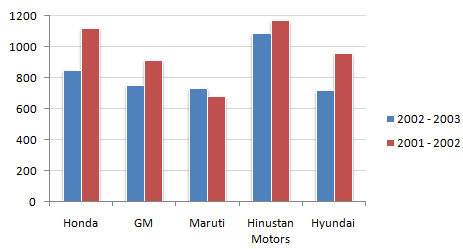# Data Interpretation - Bar Charts - Discussion

Discussion Forum : Bar Charts - Bar Chart 15 (Q.No. 1)
Directions to Solve

Study the following bar chart and answer the questions carefully.

Sales Turnover of 5 Companies (in Rs. crores)1.
What is the percentage change in the overall sales turnover of the five companies together between 2001 - 2002 and 2002 - 2003 ?
17.21 %
14.68 %
12.67 %
21.24 %
Explanation:

100 - percentage value of the fraction (Absolute change/first year's value).

Discussion:
13 comments Page 1 of 2.

Chris said:   4 years ago
There are no precise numbers, thus meaning you have to give an estimate to where the chart is?
(1)

Sharmila said:   4 years ago
Denominator value is the base value.

Base value is the one with which we compare the growth.
Here, in this case, the value has been changed from the year 2001-02 to 2002 -03.
So, 2001-02 total is taken as the base value.
(2)

Msl said:   6 years ago
How to take denominator value?

Like other bar charts, value should be given on the top of lines to calculate. Approximately, everyone has given the right calculation:

4750-4050 = 700.
(700/4750)*100 = 14.73% near to 14.68%.

Option (B).

{(4750-4050)*100}/4750 = 14.68

2001-02: approx. 1100+900+650+1150+950 = 4750
2002-03: approx. 850+750+700+1050+700 = 4050
% change = (4750-4050)*100/4750 = 14.73% (approx)Hence, chose the near one option.
Absolute value = (approx)4750-4050 = 700 (chose the near one option)
(2)

Add all the sales of both 2001-2002 and 2002-2003 separately. And find out the difference. And divide the difference by 2001-2002 add up sales. Mind it, during summation of the sales, all the sale are not exactly of round fig. i.e. it may contain 810, 840, 960 like that.

Thank you. :).

Add up all the 2001+2002 sales turnover carefully, and all the 2002+2003 values.

Than find the change percentage thereafter you will get the answer not accurately 14.68%.